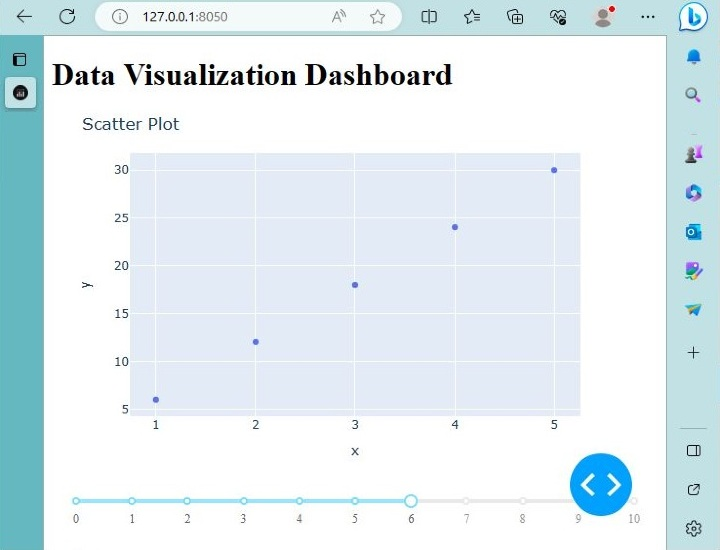# 使用Python和Plotly Dash创建基于Web的数据可视化仪表板

## 什么是Plotly Dash？

Python是一种用于数据分析和可视化的流行编程语言，提供了各种库和框架来创建交互式可视化。其中一个强大的框架就是Plotly Dash。

• Plotly Dash是一个Python框架，允许您轻松构建交互式的Web应用程序和仪表板。它结合了Python的简洁性和通用性，以及现代Web技术（如HTML、CSS和JavaScript）的灵活性和交互性。

• 借助Plotly Dash，您可以创建提供实时更新、交互式可视化和与数据源无缝集成的自定义仪表板。Plotly Dash的关键优势在于其声明性语法，这使得定义仪表板的布局和组件变得简单。

• 您可以使用Python代码描述仪表板的结构和外观，而无需编写HTML或JavaScript。这使得开发过程更快速、更易于Python开发人员访问。

• 此外，Plotly Dash提供了各种交互组件，如图形、滑块、下拉菜单和表格，可以轻松集成到您的仪表板中。这些组件使用户可以与数据进行交互，过滤和探索不同方面，并获得更深入的见解。

Plotly Dash还支持实时更新，使您的仪表板能够动态响应底层数据的变化。

## 入门

pip install dash


## 基于Web的Plotly Dash数据可视化仪表盘

import dash
import dash_core_components as dcc
import dash_html_components as html
from dash.dependencies import Input, Output


app = dash.Dash(__name__)


app.layout = html.Div(
children=[
html.H1("Data Visualization Dashboard"),
dcc.Graph(id="graph"),
dcc.Slider(
id="slider",
min=0,
max=10,
step=0.5,
value=5,
marks={i: str(i) for i in range(11)},
),
]
)


@app.callback(
Output("graph", "figure"),
[Input("slider", "value")]
)
def update_graph(value):
# Code to update the graph based on the slider value
# Replace with your own data and visualization code
# Return the updated graph figure
pass


if __name__ == "__main__":
app.run_server(debug=True)


### 示例

import dash
import dash_core_components as dcc
import dash_html_components as html
from dash.dependencies import Input, Output

app = dash.Dash(__name__)

app.layout = html.Div(
children=[
html.H1("Data Visualization Dashboard"),
dcc.Graph(id="graph"),
dcc.Slider(
id="slider",
min=0,
max=10,
step=0.5,
value=5,
marks={i: str(i) for i in range(11)},
),
]
)

@app.callback(
Output("graph", "figure"),
[Input("slider", "value")]
)
def update_graph(value):
# Placeholder code to update the graph based on the slider value
# Replace with your own data and visualization code
import plotly.express as px
import pandas as pd

# Generate sample data
df = pd.DataFrame({"x": [1, 2, 3, 4, 5], "y": [value * i for i in range(1, 6)]})

# Create a scatter plot
fig = px.scatter(df, x="x", y="y", title="Scatter Plot")

return fig

if __name__ == "__main__":
app.run_server(debug=True)


### 示例输出• 微信订阅